# TCS stock price target 2023

## How to get  tcs stock data from yahoo finance in Python?

import pandas as pd
import yfinance as yf
from statsmodels.tsa.seasonal import seasonal_decompose
start='2020-01-01',
end='2021-08-19',
progress=False)

#### What is alternative sources to get financial data?

There are number of alternative sources like Quandal,intrinio,google others.

How to plot chart Close price and Volume in python?

tcs[['Close''Volume']].plot(subplots=True, style='b',figsize=(128))
```array([<matplotlib.axes._subplots.AxesSubplot object at 0x7fc618ca3d90>,
<matplotlib.axes._subplots.AxesSubplot object at 0x7fc61745ced0>],
dtype=object)```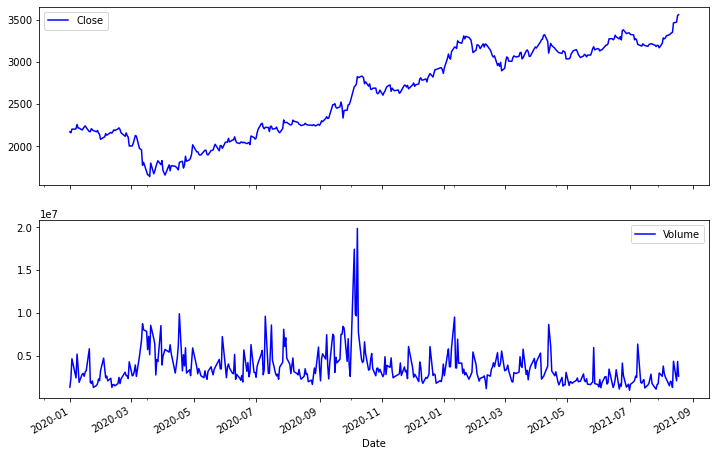tcs.describe()

### How to convert TCS Stock prices  into  log and simple returns in python?

tcs['simple_rtn'] = tcs.Close.pct_change()
tcs['log_rtn'] = np.log(tcs.Close/tcs.Close.shift(1))
tcs['log_rtn'].tail()

Date 2021-08-12 0.002255 2021-08-13 0.032768 2021-08-16 0.002754 2021-08-17 0.022802 2021-08-18 0.002109 Name: log_rtn, dtype: float64

### How to plot tcs log return in python?

tcs['log_rtn'].plot(subplots=True, style='b',
figsize=(128))
```array([<matplotlib.axes._subplots.AxesSubplot object at 0x7fc61736b590>],
dtype=object)```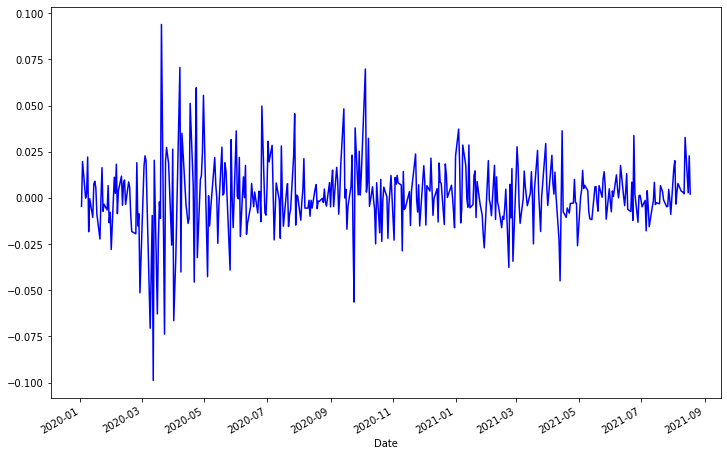#### How to change stocks frequency in python?

TCS stock price convert daily to monthly

df = df.resample('M').last()
WINDOW_SIZE = 12
df['rolling_mean'] = df.price.rolling(window=WINDOW_SIZE).mean()
df['rolling_std'] = df.price.rolling(window=WINDOW_SIZE).std()
df.plot(title='tcs Price')
Plot Tcs price with trend
`<matplotlib.axes._subplots.AxesSubplot at 0x7fc6172a89d0>`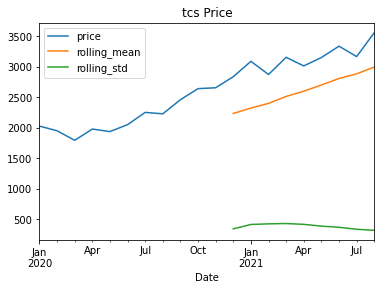### How to visualize TCS stock in 2022 with time series financial data in python?

df_future = model_prophet.make_future_dataframe(periods=365)
df_pred = model_prophet.predict(df_future)
model_prophet.plot(df_pred)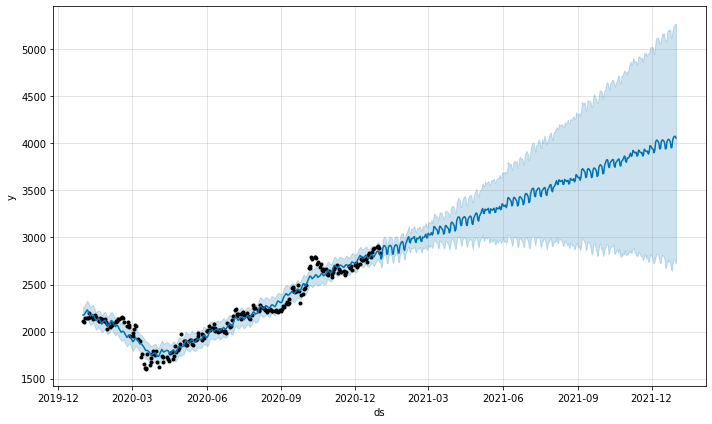model_prophet.plot_components(df_pred)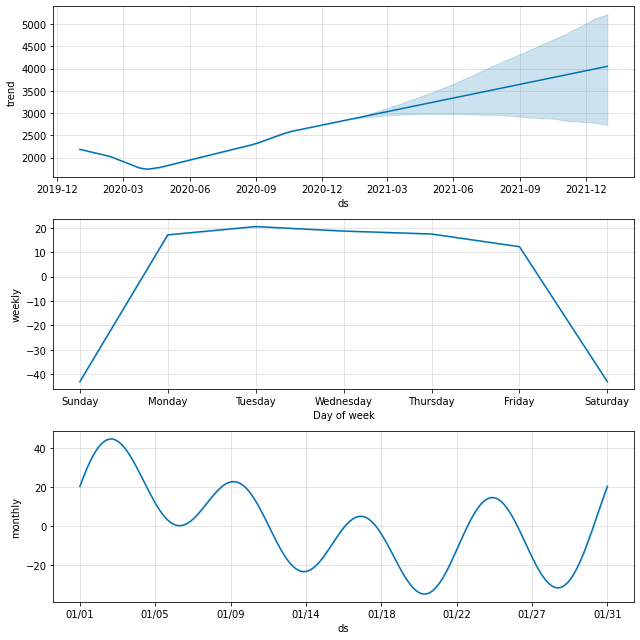How to predict vs actual Tcs stock price in 2021?

#### predicted versus actual tcs prices in 2021

selected_columns = ['ds''yhat_lower''yhat_upper''yhat']
df_pred = df_pred.loc[:, selected_columns].reset_index(drop=True)
df_test = df_test.merge(df_pred, on=['ds'], how='left')
df_test.ds = pd.to_datetime(df_test.ds)
df_test.set_index('ds', inplace=True)
fig, ax = plt.subplots(11)
ax = sns.lineplot(data=df_test[['y''yhat_lower''yhat_upper',
'yhat']])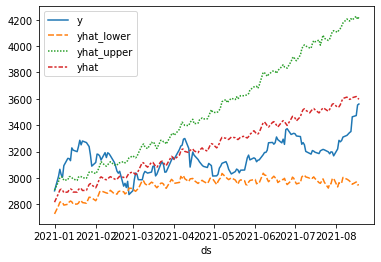fig, ax = plt.subplots(11)
ax = sns.lineplot(data=df_test[['y''yhat_lower''yhat_upper',
'yhat']])
ax.fill_between(df_test.index,
df_test.yhat_lower,
df_test.yhat_upper,
alpha=0.3)
ax.set(title='Tcs Price - actual vs. predicted',
xlabel='Date',
ylabel='TCS Price ')
```[Text(0, 0.5, 'TCS Price '),
Text(0.5, 0, 'Date'),
Text(0.5, 1.0, 'Tcs Price - actual vs. predicted')]```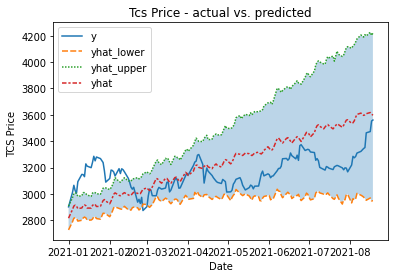### How to analyse stock p value and critical value in python?

indices = ['Test Statistic''p-value',
'# of Lags Used''# of Observations Used']
results[f'Critical Value ({key})'] = value
return results
Test Statistic -0.392382 p-value 0.911363 # of Lags Used 2.000000 # of Observations Used 17.000000 Critical Value (1%) -3.889266 dtype: float64
How to get identifying  stocks kpss in python?
def kpss_test(xh0_type='c'):
indices = ['Test Statistic''p-value''# of Lags']
kpss_test = kpss(x, regression=h0_type)
results = pd.Series(kpss_test[0:3], index=indices)
for key, value in kpss_test.items():
results[f'Critical Value ({key})'] = value
return results
kpss_test(df.price)
```The behavior of using lags=None will change in the next release. Currently lags=None is the same as lags='legacy', and so a sample-size lag length is used. After the next release, the default will change to be the same as lags='auto' which uses an automatic lag length selection method. To silence this warning, either use 'auto' or 'legacy'

```
```Test Statistic           0.360211
p-value                  0.094306
# of Lags                9.000000
Critical Value (10%)     0.347000
Critical Value (5%)      0.463000
Critical Value (2.5%)    0.574000
Critical Value (1%)      0.739000
dtype: float64```
`import statsmodels.tsa.api as smtplot_acf(df.price)plot_pacf(df.price)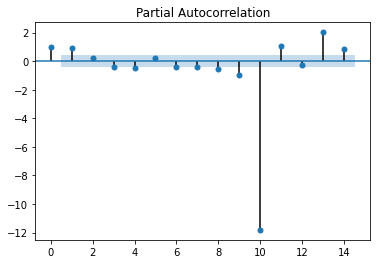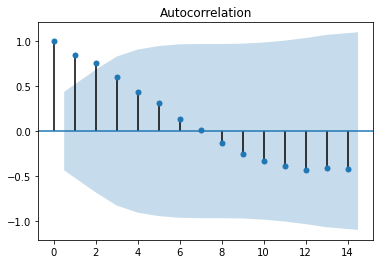`

FOR TCS UPDATE

#### For answer of these question  see other blogs

How to investigate stocks stylized facts of asset returns in python?

What is technical analysis?

The technical analysis is way to read stock price open,high,lowe and close with volume.

How to creat a candalestick chart in python?

How to apply time series modeling in stocks?

How to apply time series using facebook's prophet to get stock  value?

How to test for stationarity in time series in stocks with python?

How to correct for stationarity in time series with python?

How to use modelling time series with exponential smoothing method of stocs in python?

How to use modeling timeseris with ARIMA class model to get stocks in python?

How to get forecasting  using ARIMA class model to get stocks price in python?

How to get multi-factor models to get stock price?

How to implement the CAPM model to get stock price in python?

How to implement the Fama- French three factor model on a portfolio analys in python?

How to implement  four and five factor models in python?

What is volatility?

How to use model volatility with GARCH model in python?

How to explain stock returns volatility with ARCH models in python?

How to implement a CCC-GARCH model for multivariate volatility  forecasting in python?

How to forecast the conditional covariance matrix using DCC-GARCH to get stock price in python?

What is MonteCarlo Simulation in Finance?

How to simulate stock price dynamics using Geometric Brownian Motion in python?

How to get  stock price  American options with least squares monte carlo in python?

How to get stock ptice American Option?

How to estimate stocks value at risk using Monte Carlo in python?

How to evaluate the performance of basic 1/n portfolio in python?

How to finf the efficient frontier using Monte Carlo simulations to valu of stocks in python?

How to find the efficient frontier using optimization  stocks price with scipy in python?

How to find the efficient frontier using convex optimization stocks price with cvxpy in python?